# Graph Fundamentals

## Tree with 0+ cycle

At this point, you should be pretty familiar with trees. A tree is a special graph - a connected acyclic (cycle-less) graph. A graph may contain cycle(s) and nodes could be disconnected. A tree also contains `n` nodes and `n - 1` edges in addition to being acyclic and there exists only `1` path between `2` nodes in a tree.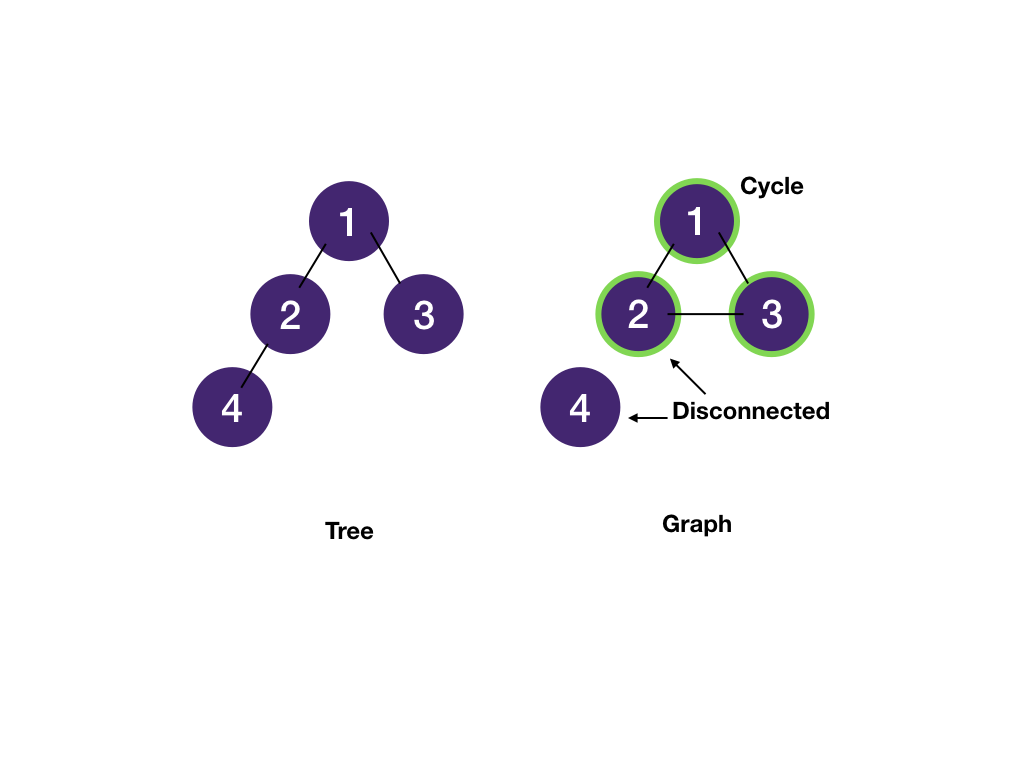## Graph Terminologies

A graph consists of vertices ("nodes" in trees) and edges. Vertices are connected by edges. Two vertices connected by an edge are called neighbors and are adjacent ("children" and "parents" in trees).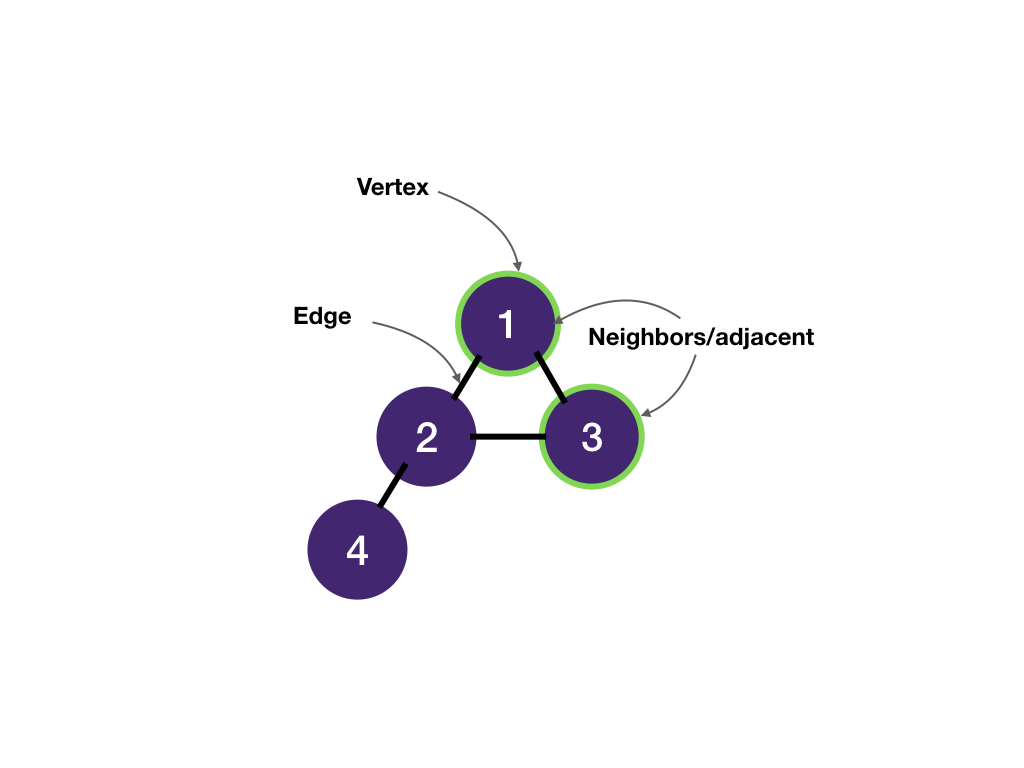Edges can be undirected or directed. For most interview problems, we are dealing with undirected graphs. A tree is also an undirected graph. We'll discuss directed graph in the course schedule module.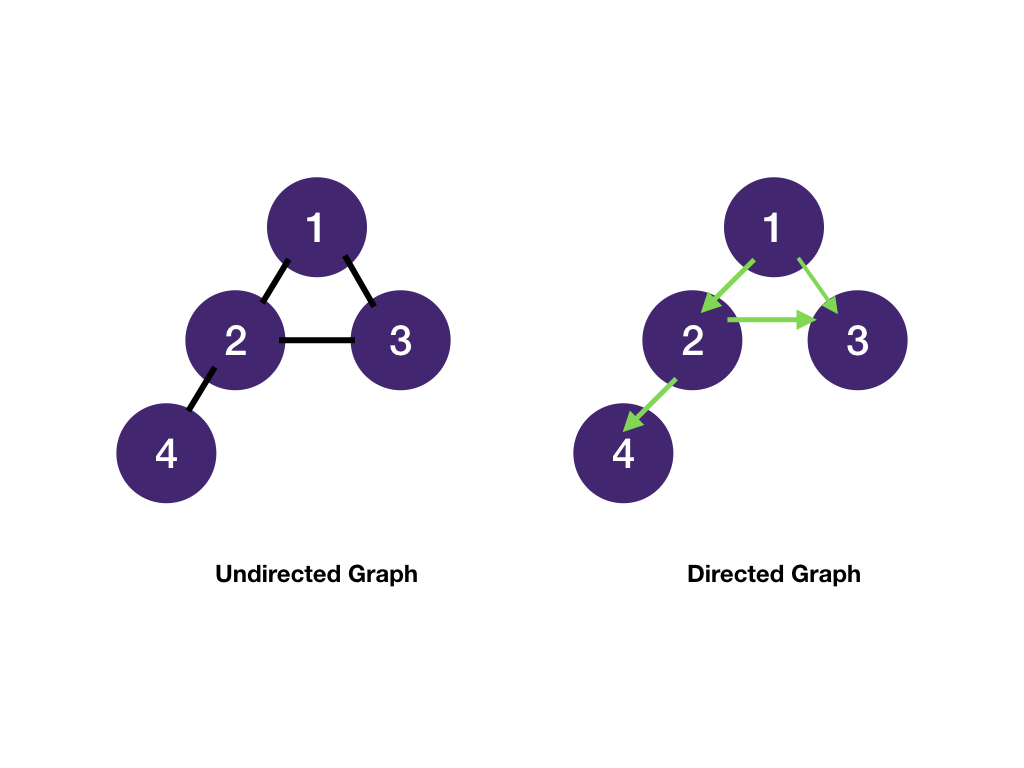A path is a sequence of vertices. A cycle is a path that starts and ends at the same vertex.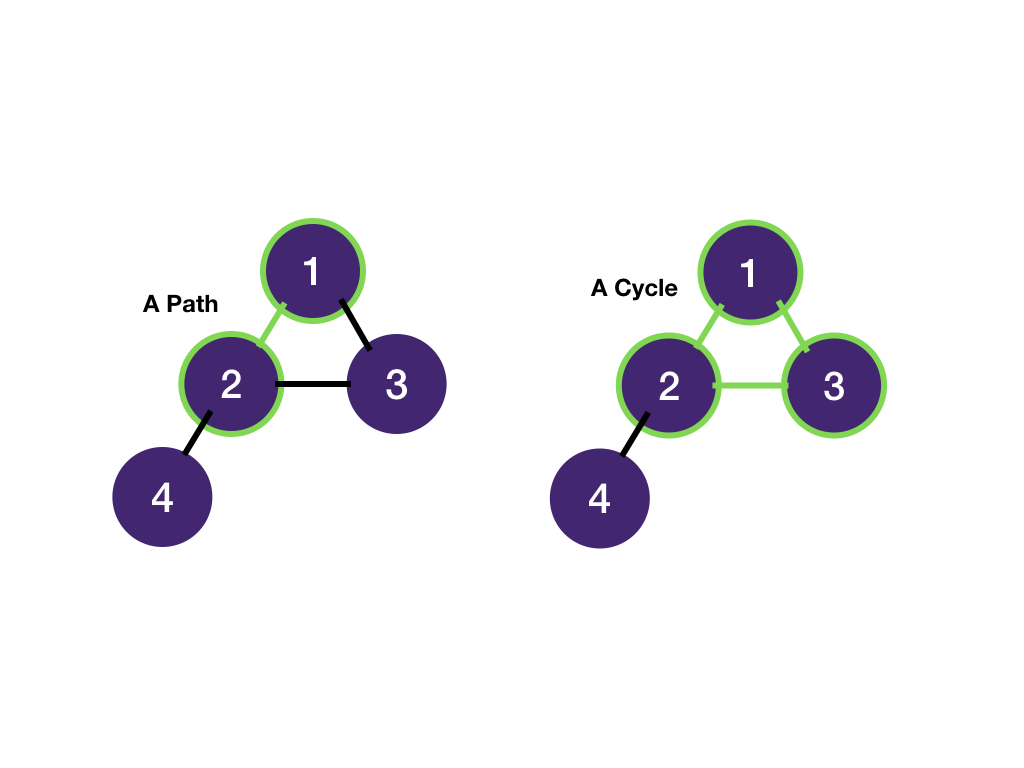An undirected graph is connected if every vertex is joined by a path to another vertex. Otherwise, it's disconnected.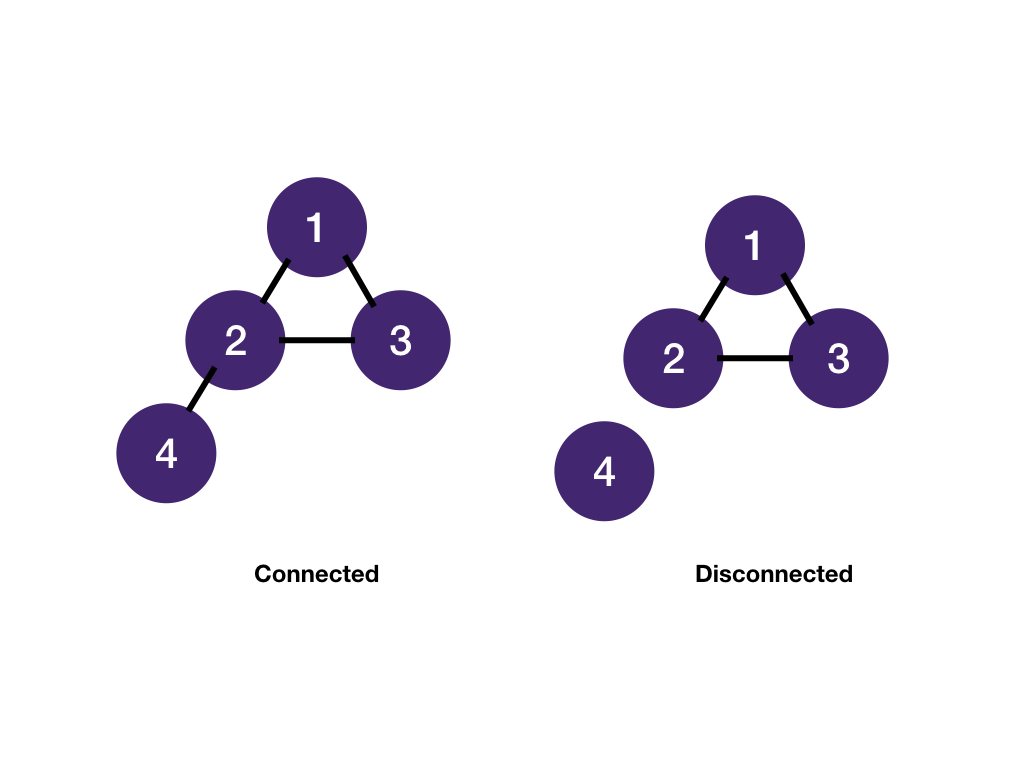A graph is most commonly stored as a map of adjacency lists: for each vertex, store a list of its neighbors.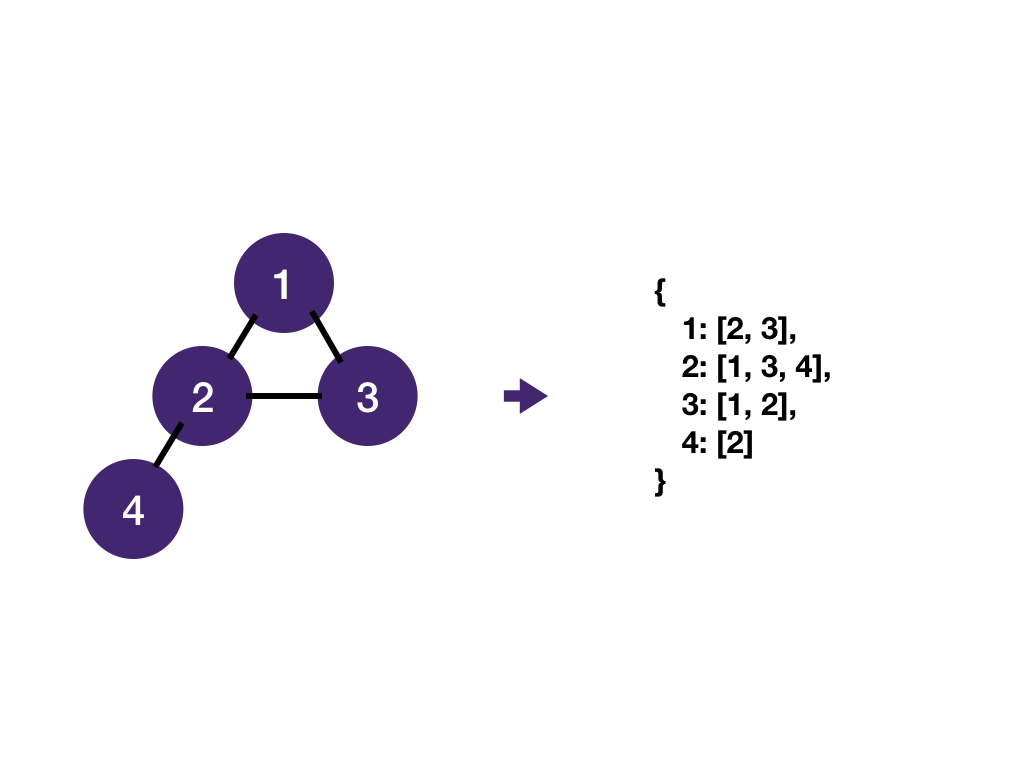Note that even though a graph is represented as an adjacency list , we don't actually have to create it upfront. What we really need is a function to get a vertex's neighbors. We'll see that in the next module.

Still not clear? Submit the part you don't understand to our editors.